## AVL Tree-

Before you go through this article, make sure that you have gone through the previous article on AVL Trees.

We have discussed-

• AVL trees are self-balancing binary search trees.
• In AVL trees, balancing factor of each node is either 0 or 1 or -1.

## AVL Tree Properties-

Important properties of AVL tree are-

## Property-01:

 Maximum possible number of nodes in AVL tree of height H= 2H+1 – 1

### Example-

Maximum possible number of nodes in AVL tree of height-3

= 23+1 – 1

= 16 – 1

= 15

Thus, in AVL tree of height-3, maximum number of nodes that can be inserted = 15.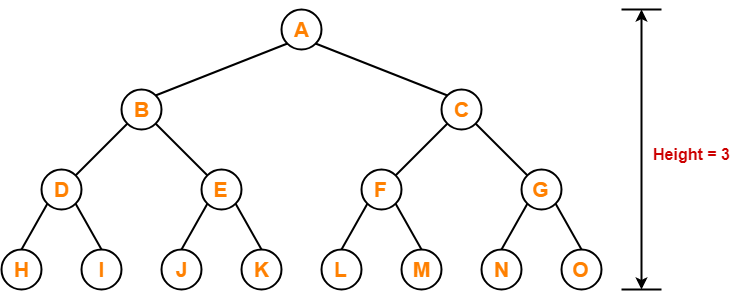We can not insert more number of nodes in this AVL tree.

## Property-02:

 Minimum number of nodes in AVL Tree of height H is given by a recursive relation-N(H) = N(H-1) + N(H-2) + 1

Base conditions for this recursive relation are-

• N(0) = 1
• N(1) = 2

### Example-

Minimum possible number of nodes in AVL tree of height-3 = 7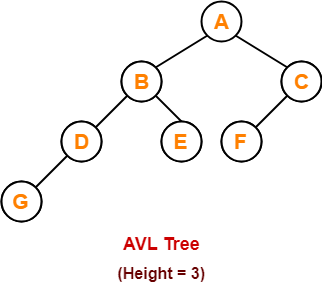(For explanation, Refer problem-01)

## Property-03:

 Minimum possible height of AVL Tree using N nodes= ⌊log2N⌋

### Example-

Minimum possible height of AVL Tree using 8 nodes

= ⌊log28⌋

= ⌊log223

= ⌊3log22⌋

= ⌊3⌋

= 3

## Property-04:

 Maximum height of AVL Tree using N nodes is calculated using recursive relation-N(H) = N(H-1) + N(H-2) + 1

Base conditions for this recursive relation are-

• N(0) = 1
• N(1) = 2

## NOTE-

• If there are n nodes in AVL Tree, its maximum height can not exceed 1.44log2n.
• In other words, Worst case height of AVL Tree with n nodes = 1.44log2n.

## Problem-01:

Find the minimum number of nodes required to construct AVL Tree of height = 3.

## Solution-

We know, minimum number of nodes in AVL tree of height H is given by a recursive relation-

 N(H) = N(H-1) + N(H-2) + 1

where N(0) = 1 and N(1) = 2

### Step-01:

Substituting H = 3 in the recursive relation, we get-

N(3) = N(3-1) + N(3-2) + 1

N(3) = N(2) + N(1) + 1

N(3) = N(2) + 2 + 1                (Using base condition)

N(3) = N(2) + 3                      …………(1)

To solve this recursive relation, we need the value of N(2).

### Step-02:

Substituting H = 2 in the recursive relation, we get-

N(2) = N(2-1) + N(2-2) + 1

N(2) = N(1) + N(0) + 1

N(2) = 2 + 1 + 1                 (Using base conditions)

∴ N(2) = 4                          …………(2)

### Step-03:

Using (2) in (1), we get-

N(3) = 4 + 3

∴ N(3) = 7

Thus, minimum number of nodes required to construct AVL tree of height-3 = 7.

## Problem-02:

Find the minimum number of nodes required to construct AVL Tree of height = 4.

## Solution-

We know, minimum number of nodes in AVL tree of height H is given by a recursive relation-

 N(H) = N(H-1) + N(H-2) + 1

where N(0) = 1 and N(1) = 2

### Step-01:

Substituting H = 4 in the recursive relation, we get-

N(4) = N(4-1) + N(4-2) + 1

N(4) = N(3) + N(2) + 1               …………(1)

To solve this recursive relation, we need the value of N(2) and N(3).

### Step-02:

Substituting H = 2 in the recursive relation, we get-

N(2) = N(2-1) + N(2-2) + 1

N(2) = N(1) + N(0) + 1

N(2) = 2 + 1 + 1                 (Using base conditions)

∴ N(2) = 4                          …………(2)

### Step-03:

Substituting H = 3 in the recursive relation, we get-

N(3) = N(3-1) + N(3-2) + 1

N(3) = N(2) + N(1) + 1

N(3) = 4 + 2 + 1                 (Using (2) and base condition)

∴ N(3) = 7                          …………(3)

### Step-04:

Using (2) and (3) in (1), we get-

N(4) = 7 + 4 + 1

∴ N(4) = 12

Thus, minimum number of nodes required to construct AVL tree of height-4 = 12.

## Problem-03:

What is the maximum height of any AVL tree with 10 nodes?

## Solution-

For calculating the maximum height of AVL tree with n nodes, we use a recursive relation-

 N(H) = N(H-1) + N(H-2) + 1

### Step-01:

Substituting H = 2 in the recursive relation, we get-

N(2) = N(2-1) + N(2-2) + 1

N(2) = N(1) + N(0) + 1

N(2) = 2 + 1 + 1                (Using base conditions)

∴ N(2) = 4                          …………(1)

So, minimum number of nodes required to construct AVL tree of height-2 = 4.

### Step-02:

Substituting H = 3 in the recursive relation, we get-

N(3) = N(3-1) + N(3-2) + 1

N(3) = N(2) + N(1) + 1

N(3) = 4 + 2 + 1                (Using (1) and base condition)

∴ N(3) = 7                          …………(2)

So, minimum number of nodes required to construct AVL tree of height-3 = 7.

### Step-03:

Substituting H = 4 in the recursive relation, we get-

N(4) = N(4-1) + N(4-2) + 1

N(4) = N(3) + N(2) + 1

N(4) = 7 + 4 + 1                (Using (1) and (2))

∴ N(4) = 12

So, minimum number of nodes required to construct AVL tree of height-4 = 12.

But given number of nodes = 10 which is less than 12.

Thus, maximum height of AVL tree that can be obtained using 10 nodes = 3.

## Problem-04:

What is the maximum height of any AVL tree with 77 nodes?

## Solution-

For calculating the maximum height of AVL tree with n nodes, we use a recursive relation-

 N(H) = N(H-1) + N(H-2) + 1

### Step-01:

Substituting H = 2 in the recursive relation, we get-

N(2) = N(2-1) + N(2-2) + 1

N(2) = N(1) + N(0) + 1

N(2) = 2 + 1 + 1                (Using base conditions)

∴ N(2) = 4                          …………(1)

So, minimum number of nodes required to construct AVL tree of height-2 = 4.

### Step-02:

Substituting H = 3 in the recursive relation, we get-

N(3) = N(3-1) + N(3-2) + 1

N(3) = N(2) + N(1) + 1

N(3) = 4 + 2 + 1                (Using (1) and base condition)

∴ N(3) = 7                          …………(2)

So, minimum number of nodes required to construct AVL tree of height-3 = 7.

### Step-03:

Substituting H = 4 in the recursive relation, we get-

N(4) = N(4-1) + N(4-2) + 1

N(4) = N(3) + N(2) + 1

N(4) = 7 + 4 + 1                (Using (1) and (2))

∴ N(4) = 12                        …………(3)

So, minimum number of nodes required to construct AVL tree of height-4 = 12.

### Step-04:

Substituting H = 5 in the recursive relation, we get-

N(5) = N(5-1) + N(5-2) + 1

N(5) = N(4) + N(3) + 1

N(5) = 12 + 7 + 1                (Using (2) and (3))

∴ N(5) = 20                          …………(4)

So, minimum number of nodes required to construct AVL tree of height-5 = 20.

### Step-05:

Substituting H = 6 in the recursive relation, we get-

N(6) = N(6-1) + N(6-2) + 1

N(6) = N(5) + N(4) + 1

N(6) = 20 + 12 + 1                (Using (3) and (4))

∴ N(6) = 33                            …………(5)

So, minimum number of nodes required to construct AVL tree of height-6 = 33.

### Step-06:

Substituting H = 7 in the recursive relation, we get-

N(7) = N(7-1) + N(7-2) + 1

N(7) = N(6) + N(5) + 1

N(7) = 33 + 20 + 1                (Using (4) and (5))

∴ N(7) = 54                            …………(6)

So, minimum number of nodes required to construct AVL tree of height-7 = 54.

### Step-07:

Substituting H = 8 in the recursive relation, we get-

N(8) = N(8-1) + N(8-2) + 1

N(8) = N(7) + N(6) + 1

N(8) = 54 + 33 + 1                (Using (5) and (6))

∴ N(8) = 88                            …………(6)

So, minimum number of nodes required to construct AVL tree of height-8 = 88.

But given number of nodes = 77 which is less than 88.

Thus, maximum height of AVL tree that can be obtained using 77 nodes = 7.

Next Article- Insertion in AVL Tree

Get more notes and other study material of Data Structures.

Watch video lectures by visiting our YouTube channel LearnVidFun.

## AVL Tree-

Before you go through this article, make sure that you have gone through the previous article on AVL Trees.

We have discussed-

• AVL trees are self-balancing binary search trees.
• In AVL trees, balancing factor of each node is either 0 or 1 or -1.

## Insertion in AVL Tree-

 Insertion Operation is performed to insert an element in the AVL Tree.

To insert an element in the AVL tree, follow the following steps-

• Insert the element in the AVL tree in the same way the insertion is performed in BST.
• After insertion, check the balance factor of each node of the resulting tree.

Read More- Insertion in Binary Search Tree

Now, following two cases are possible-

### Case-01:

• After the insertion, the balance factor of each node is either 0 or 1 or -1.
• In this case, the tree is considered to be balanced.
• Conclude the operation.
• Insert the next element if any.

### Case-02:

• After the insertion, the balance factor of at least one node is not 0 or 1 or -1.
• In this case, the tree is considered to be imbalanced.
• Perform the suitable rotation to balance the tree.
• After the tree is balanced, insert the next element if any.

## Rule-01:

After inserting an element in the existing AVL tree,

• Balance factor of only those nodes will be affected that lies on the path from the newly inserted node to the root node.

## Rule-02:

To check whether the AVL tree is still balanced or not after the insertion,

• There is no need to check the balance factor of every node.
• Check the balance factor of only those nodes that lies on the path from the newly inserted node to the root node.

## Rule-03:

After inserting an element in the AVL tree,

• If tree becomes imbalanced, then there exists one particular node in the tree by balancing which the entire tree becomes balanced automatically.
• To re balance the tree, balance that particular node.

To find that particular node,

• Traverse the path from the newly inserted node to the root node.
• Check the balance factor of each node that is encountered while traversing the path.
• The first encountered imbalanced node will be the node that needs to be balanced.

To balance that node,

• Count three nodes in the direction of leaf node.
• Then, use the concept of AVL tree rotations to re balance the tree.

## Problem-

Construct AVL Tree for the following sequence of numbers-

50 , 20 , 60 , 10 , 8 , 15 , 32 , 46 , 11 , 48

## Solution-

### Step-01: Insert 50### Step-02: Insert 20

• As 20 < 50, so insert 20 in 50’s left sub tree.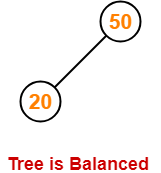### Step-03: Insert 60

• As 60 > 50, so insert 60 in 50’s right sub tree.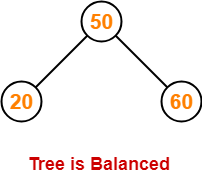### Step-04: Insert 10

• As 10 < 50, so insert 10 in 50’s left sub tree.
• As 10 < 20, so insert 10 in 20’s left sub tree.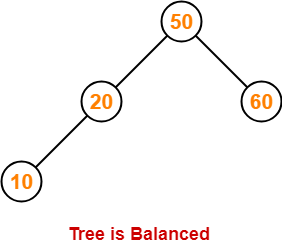### Step-05: Insert 8

• As 8 < 50, so insert 8 in 50’s left sub tree.
• As 8 < 20, so insert 8 in 20’s left sub tree.
• As 8 < 10, so insert 8 in 10’s left sub tree.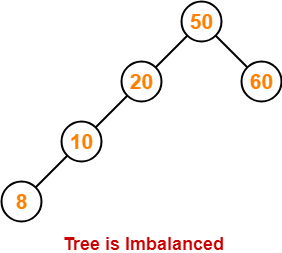To balance the tree,

• Find the first imbalanced node on the path from the newly inserted node (node 8) to the root node.
• The first imbalanced node is node 20.
• Now, count three nodes from node 20 in the direction of leaf node.
• Then, use AVL tree rotation to balance the tree.

Following this, we have-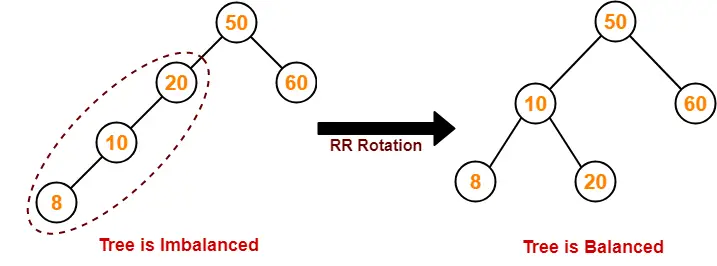### Step-06: Insert 15

• As 15 < 50, so insert 15 in 50’s left sub tree.
• As 15 > 10, so insert 15 in 10’s right sub tree.
• As 15 < 20, so insert 15 in 20’s left sub tree.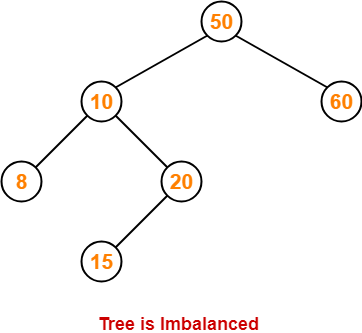To balance the tree,

• Find the first imbalanced node on the path from the newly inserted node (node 15) to the root node.
• The first imbalanced node is node 50.
• Now, count three nodes from node 50 in the direction of leaf node.
• Then, use AVL tree rotation to balance the tree.

Following this, we have-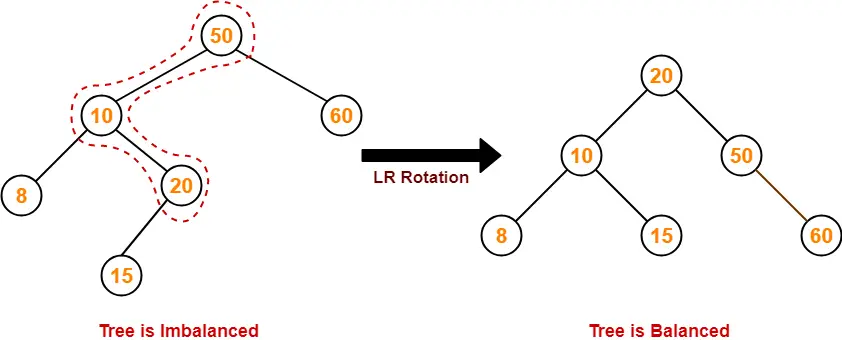### Step-07: Insert 32

• As 32 > 20, so insert 32 in 20’s right sub tree.
• As 32 < 50, so insert 32 in 50’s left sub tree.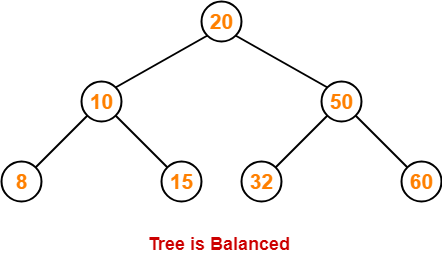### Step-08: Insert 46

• As 46 > 20, so insert 46 in 20’s right sub tree.
• As 46 < 50, so insert 46 in 50’s left sub tree.
• As 46 > 32, so insert 46 in 32’s right sub tree.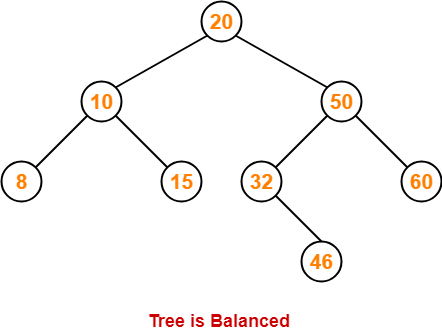### Step-09: Insert 11

• As 11 < 20, so insert 11 in 20’s left sub tree.
• As 11 > 10, so insert 11 in 10’s right sub tree.
• As 11 < 15, so insert 11 in 15’s left sub tree.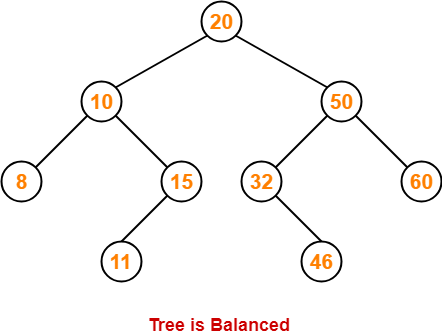### Step-10: Insert 48

• As 48 > 20, so insert 48 in 20’s right sub tree.
• As 48 < 50, so insert 48 in 50’s left sub tree.
• As 48 > 32, so insert 48 in 32’s right sub tree.
• As 48 > 46, so insert 48 in 46’s right sub tree.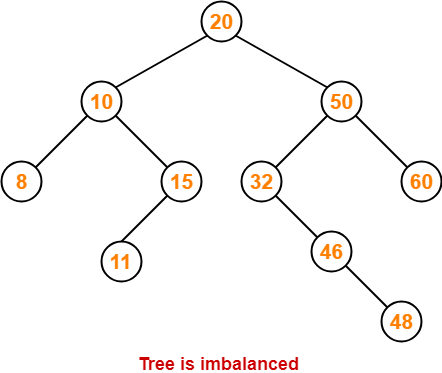To balance the tree,

• Find the first imbalanced node on the path from the newly inserted node (node 48) to the root node.
• The first imbalanced node is node 32.
• Now, count three nodes from node 32 in the direction of leaf node.
• Then, use AVL tree rotation to balance the tree.

Following this, we have-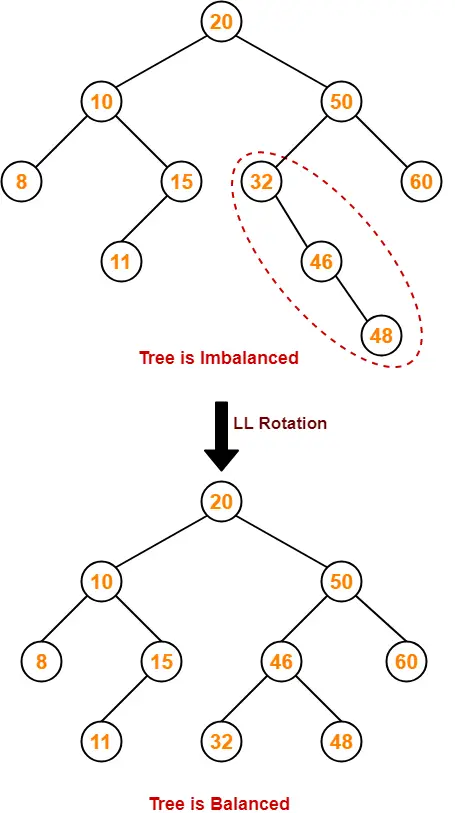This is the final balanced AVL tree after inserting all the given elements.

To gain better understanding of AVL Tree Insertion,

Watch this Video Lecture

Next Article- Heap Data Structure

Get more notes and other study material of Data Structures.

Watch video lectures by visiting our YouTube channel LearnVidFun.

## AVL Tree-

• AVL trees are special kind of binary search trees.
• In AVL trees, height of left subtree and right subtree of every node differs by at most one.
• AVL trees are also called as self-balancing binary search trees.

## Example-

Following tree is an example of AVL tree-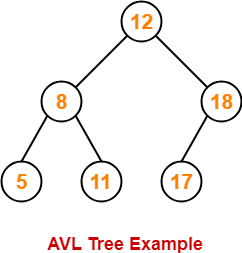This tree is an AVL tree because-

• It is a binary search tree.
• The difference between height of left subtree and right subtree of every node is at most one.

Following tree is not an example of AVL Tree-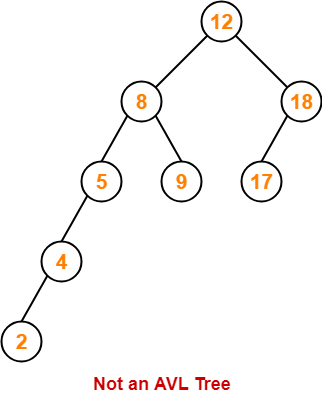This tree is not an AVL tree because-

• The difference between height of left subtree and right subtree of root node = 4 – 2 = 2.
• This difference is greater than one.

## Balance Factor-

In AVL tree,

• Balance factor is defined for every node.
• Balance factor of a node = Height of its left subtree – Height of its right subtree

 In AVL tree,Balance factor of every node is either 0 or 1 or -1.

## AVL Tree Operations-

Like BST Operations, commonly performed operations on AVL tree are-

1. Search Operation
2. Insertion Operation
3. Deletion Operation

Also Read- Insertion in AVL Tree

After performing any operation on AVL tree, the balance factor of each node is checked.

There are following two cases possible-

### Case-01:

• After the operation, the balance factor of each node is either 0 or 1 or -1.
• In this case, the AVL tree is considered to be balanced.
• The operation is concluded.

### Case-02:

• After the operation, the balance factor of at least one node is not 0 or 1 or -1.
• In this case, the AVL tree is considered to be imbalanced.
• Rotations are then performed to balance the tree.

## AVL Tree Rotations-

 Rotation is the process of moving the nodes to make tree balanced.

## Kinds of Rotations-

There are 4 kinds of rotations possible in AVL Trees-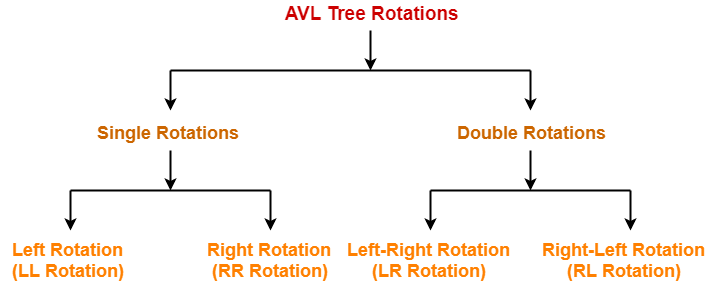1. Left Rotation (LL Rotation)
2. Right Rotation (RR Rotation)
3. Left-Right Rotation (LR Rotation)
4. Right-Left Rotation (RL Rotation)

### Case-01: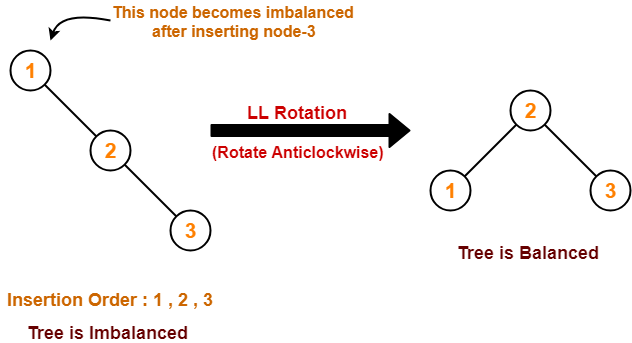### Case-02: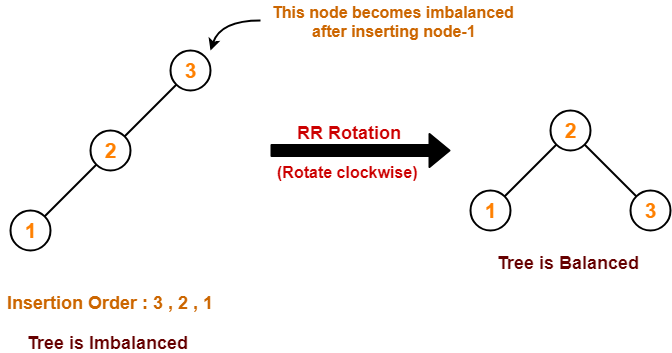### Case-03: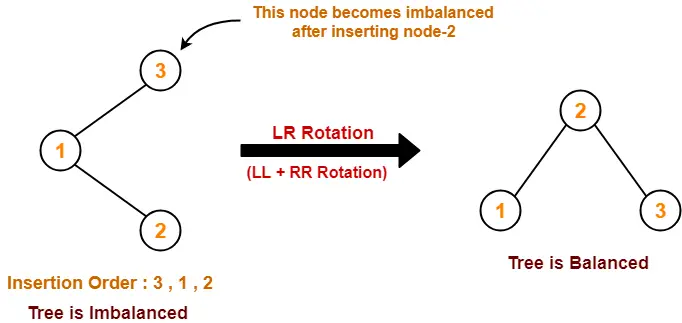### Case-04: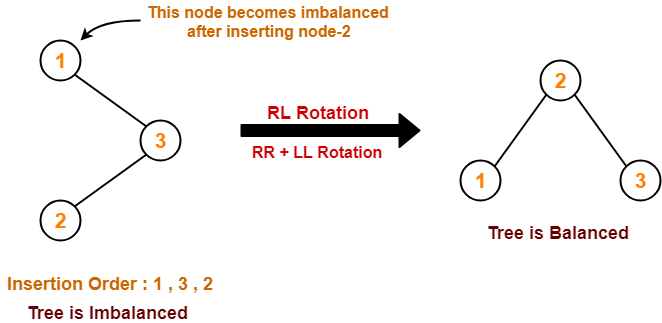To gain better understanding about AVL Trees and Rotations,

Watch this Video LectureNext Article- AVL Tree Properties

Get more notes and other study material of Data Structures.

Watch video lectures by visiting our YouTube channel LearnVidFun.